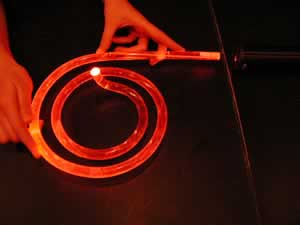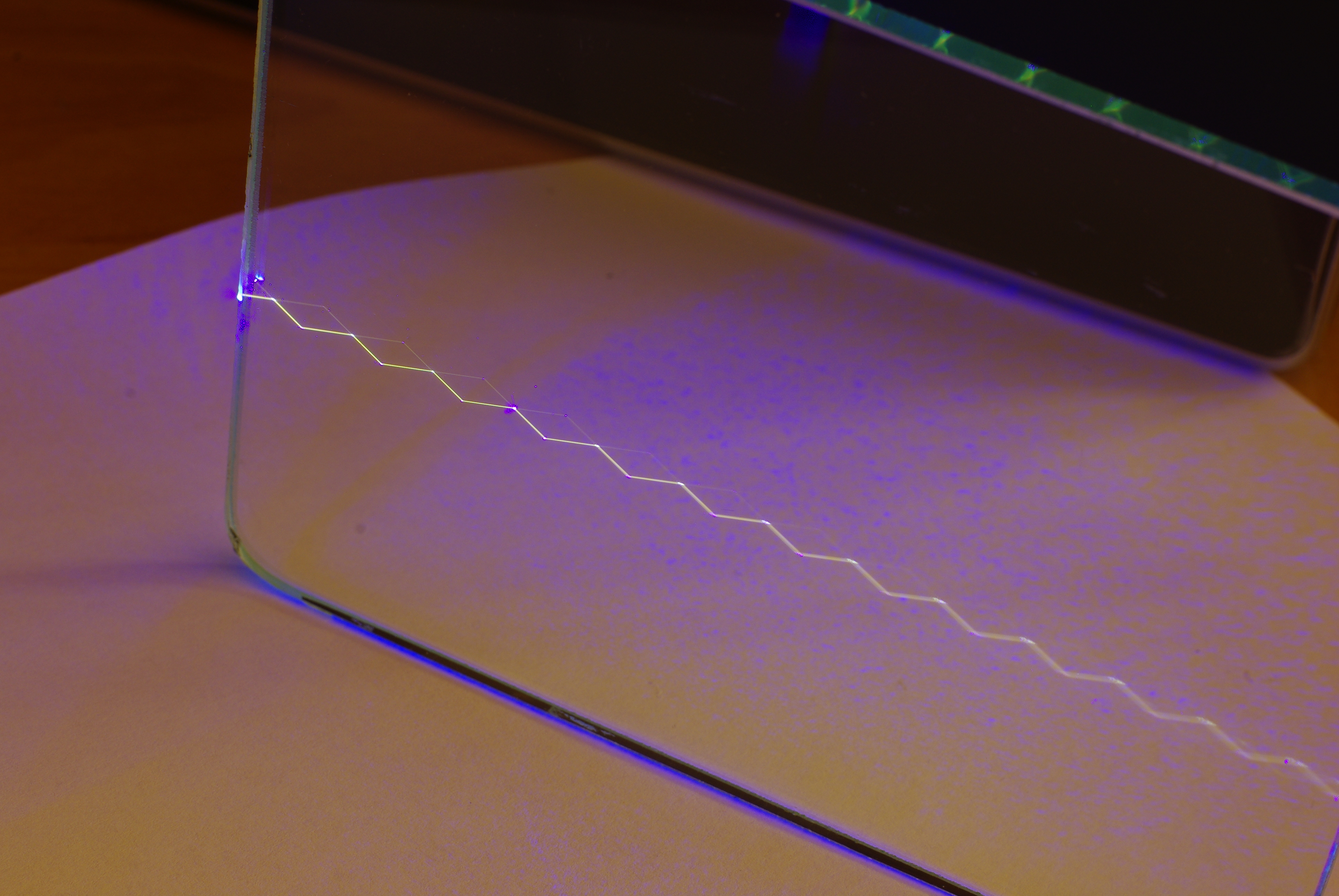# Lecture 24: Light: Reflection and Refraction

## index of refraction

• In the material the light always moves with speed v that is slower than in vacuum.
• The ration n = c/v is called index of refraction and is one of the most important optical properties of a material. Typical values for glasses are n ~ 1.5-2 while for the air it is 1.0003, which is practically unity
• Index of refraction is always larger than unity n > 1 , equal to unity only in vacuum.

## Refraction and reflection

Two fundamental optical phenomena - reflection and refraction - happen at the interface between two materials with different index of refraction n

## Reflection

• Light is reflected is reflected from any surface (excluding the perfect black body where all incident light is absorbed).
• From the rough surface light is reflected in all directions, we deal with diffuse reflection
• We shall be interested in sufficiently smooth (polished) surfaces , when incident plane wave is reflected as an approximately plane wave again. Thus reflected light can be described by a ray.

The law of reflection: angle between the incident ray and the normal to the surface is the same as the angle between reflected ray and the normal

θr = θa

## Properties of reflection

• Path is reversable
• Frequency and wavelength of reflected light are unchanged
• Intensity of reflected is not equal to the intensity of the incident light. Some light is absorbed even by the best mirrors. How much is reflected depends on properties of the mirror, wavelength of light and light polarization
• Intensity of reflected light increases with the angle.
• Reflected from two mirrors at right angle, the light goes directly back for any incident angle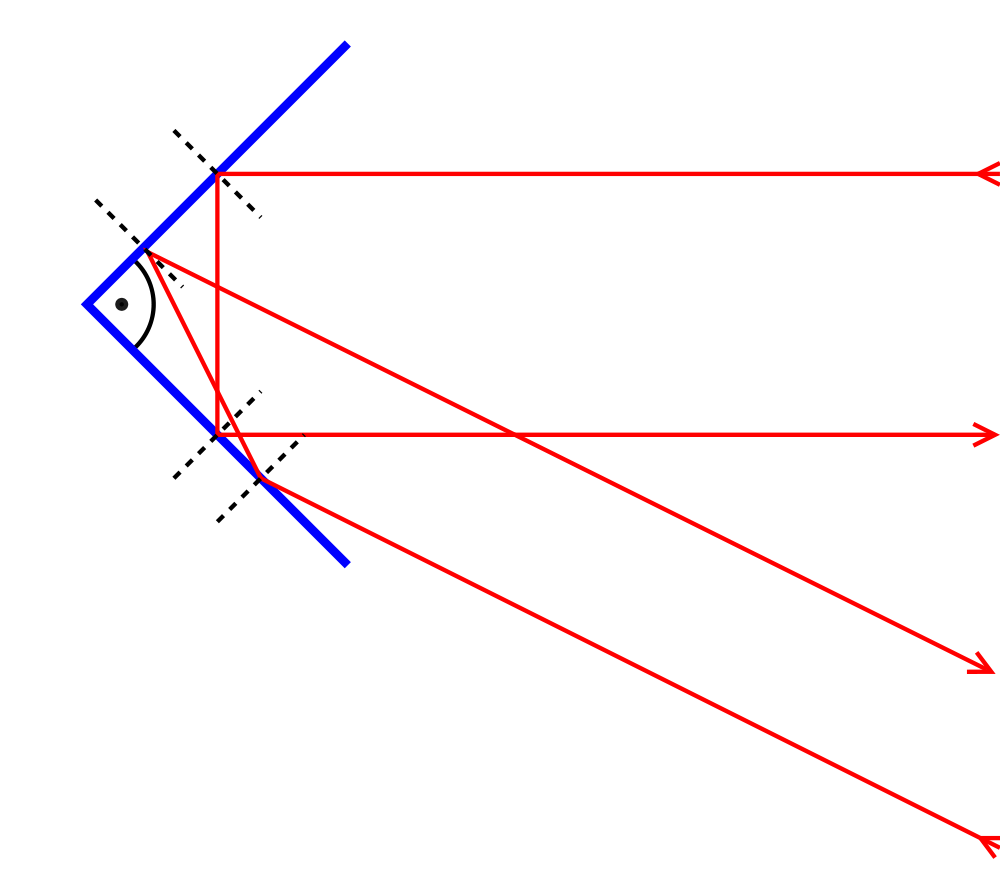## Refraction

• When light passes through surface interface between two transparent materials with different refaction indexes n it exhibits both reflection and refraction - change of direction of propagation

The law of refaction: angle between the incident ray and the normal to the surface and the angle between transmitted ray and the normal are in relation

Snell's Law:        na sin θa = nb sin θb

## Properties

• The refracted path is reversable. If we send the light from the other end, it will follow the same path (but reflected ray will be different)
• The frequency of light remains unchanged in both mediums. But the wavelengths changes λa / λ = nb / na
• Distribution of intensity in reflected and transimitted beams depends on refraction indexes, frequency, polarization of light and the angle
• More light is reflected and less transmitted at higher incident angles

## Brief explanation of refraction effect

• One should not think of the light ray as a narrow line where there is light. Ray just represents the normal to wavefront, the light intensity is appreciable over some beam width D , much wider than the wavelength D > λ .
• If we had an exact plane wave, it would extend in the planes orthogonal to the ray all the way to infinity in all directions !
 The main cause of the refraction is that the part of the wave that enters the material with another index of refraction changes its wave speed If light hits the interface under an angle, the point of contact moves from one edge of the wavefront to another, and so is the moment when a segment of wavefront changes speed. This causes the wavefront (and the light ray) to turn.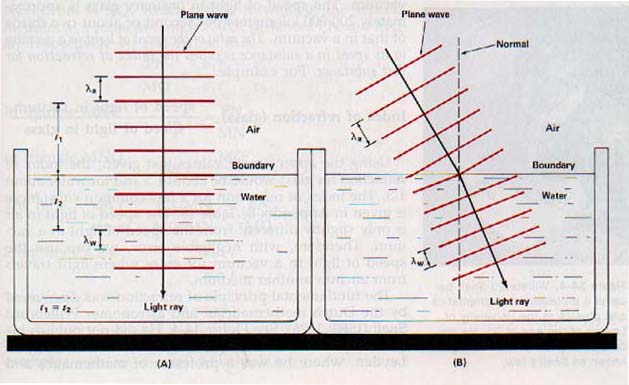## Total internal refraction: prisms, waveguides

• The sin of the angle of refracted ray according to Snell's Law is
sin θb = ( na / nb ) sin θa
• But it must be less than unity. When light travels from the medium with smaller na to larger nb (air to glass), this is possible for any incident angles θa . There is always a transmitted ray
• However when light travels from the medium with larger na to smaller nb (glass to air), there is a critical incident angle
sin θcrit = nb / na < 1
exceeding which this is not possible !
• for θ > θcrit there is no trasmitted beam. We have a total reflection. It is called total internal reflection because it is usually inside the material.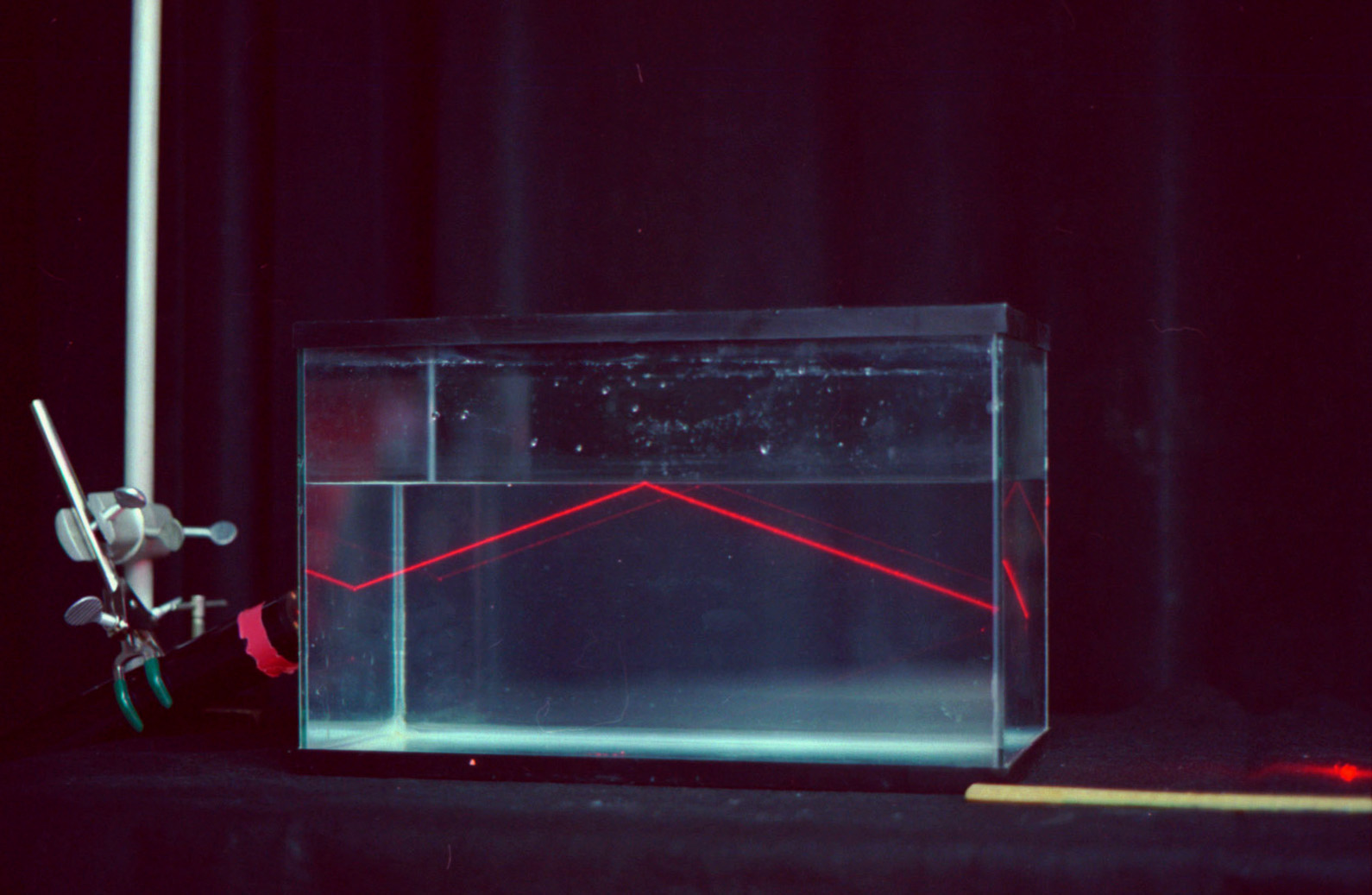• Total internal reflection has multiple technological uses: photographics lenses/prisms, optical waveguides/fiberoptics etc.